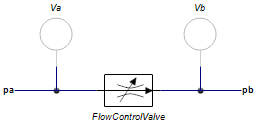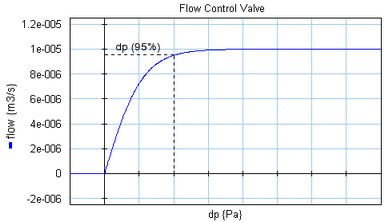﻿ 20-sim webhelp > Library > Iconic Diagrams > Hydraulics > Valves > flowcontrolvalve-states

# flowcontrolvalve-states

## Library

Iconic Diagrams\Hydraulics\Valves\Basic Valves

## Use

Domains: Continuous. Size: 1-D. Kind: Iconic Diagrams (Hydraulics).

## DescriptionThis model describes a flow control valve with with parasitic volumes.In this model the flow is modeled by an tanh function. The pressure drop is defined as the pressure where 95% of the desired flow rate is achieved.

dp = pa.p - pb.p

phi = if dp > 0 then

Q_set*tanh( (arctan(0.95)/p_drop) * dp) + GLeak * dp;

else

GLeak * dp

end;

Here phi is the desired flow and p_drop is the 95% pressure drop. Gleak is the conductance of laminar leakage flow when the valve is closed. The pressure at both ports has a lower limit that is equal to the vapour pressure. Therefore the actual equations used in this model are:

p1_lim = if pa.p < p_vapour then p_vapour else pa.p end;

p2_lim = if pb.p < p_vapour then p_vapour else pb.p end;

dp = p1_lim - p2_lim;

## Interface

 Ports Description pa, pb Both terminals of the hydraulic component. Causality fixed volume flow out pa fixed volume flow out pb Parameters FlowControlValve\Q_set FlowControlValve\p_drop FlowControlValve\GLeak Va\V Va\B Va\p_initial Vb\V Vb\B Vb\p_initial Desired flow [m3/s] pressure drop at 95% flow [Pa] Conductance of the laminar leakage flow [m3/s.Pa], GLeak >= 0! Volume of oil under pressure [m3] (hidden) Effective bulk modulus [Pa] (hidden) The starting pressure of the volume [Pa] (hidden) Volume of oil under pressure [m3] (hidden) Effective bulk modulus [Pa] (hidden) The starting pressure of the volume [Pa] (hidden)